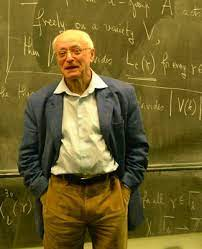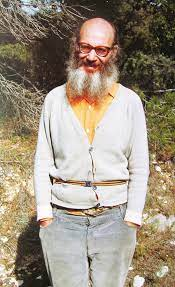# Geometric class field theory (Spring 2022)

Geometric class field theory began as the study of class field theory for function fields using algebraic geometry. This was done by Rosenlicht and Lang through the construction and study of generalized Jacobians. New phenomena arise, such as the theory over additional base fields. This work was further clarified and extended in Serre's book [S]. Since then, Deligne and others have reformulated this theory to be part of geometric Langlands. Less well-known is Grothendieck's program, sketched in letters to Serre and Breen [G1], [G2]. Grothendieck gives several extensions of geometric class field theory through duality, derived categories, to a relative setting, and more. This proposal has not ben much pursued; the main exception is the work of Contou-Carrère [C1, C2], one of Grothendieck's later students. In this seminar we will review the basic theory as in [S] and write it down using scheme theory. Then we will try to work out parts of Grothendieck's proposal.
Note: due to the difficulty of the proposed topic, the last few weeks of the seminar ended up being on various topics in arithmetic geometry.

• Organizer: Caleb Ji
• When: Wednesday 12:45 pm - 1:45 pm
• Where: Math 622
• References:
[C2] C. Contou-Carrère, Jacobiennes globales relatives, in: The Grothendieck Festschrift, Vol. II, Progr. Math. 87, Birkhauser, Boston 1990, P. Cartier, et al., eds., 69–109
[C2] C. Contou-Carrère, Jacobienne locale d'une courbe formelle relative, Rendiconti del Seminario Matematico della Università di Padova 130 (2013), 1-106.
[S] J. P. Serre, Algebraic Groups and Class Fields, Springer-Verlag NY (1988).
[G1] A. Grothendieck, Grothendieck-Serre Correspondence Letter of August 9, 1960.
[G2] A. Grothendieck, Appendix to Pursuing Stacks, here.
• Notes from the seminar are not here.

#### Schedule

Feb 9
Caleb Ji
Organizational meeting
We will discuss the plan for this seminar and give a rough outline of the main ideas to be used. We will also see how Jacobians can be used to study abelian coverings of curves.
Feb 16
Caleb Ji
Artin-Verdier duality Notes
Artin-Verdier duality is a duality theorem for the étale cohomology of curves. I will outline a proof of this theorem, which involves dévissage arguments and class field theory. Then I will speculate on a possible common generalization of this theorem and geometric class field theory proposed by Grothendieck.
Feb 23
Caleb Ji
Grothendieck's new duality formula I
In a letter to Breen [G2], Grothendieck proposes a new duality formula which specializes to both geometric class field theory and arithmetic class field theory. He also explains a local version. We will discuss this.
Mar 2
Caleb Ji
Grothendieck's new duality formula II
Last week we discussed the meaning of Grothendieck's proposed duality formula in the case of a curve and unequal characteristic. This case is already known, but there are links between it and Serre's description of geometric class field theory that we will discuss this week. If time permits, we will examine the equal characteristic case.
Mar 9
Caleb Ji
Serre's geometric class field theory
Grothendieck has proposed a duality formula which recovers Serre's geometric class field theory in a special case. This week we will examine this case in detail and unwind the statements carefully to see why this is.
Mar 16
Spring break
Mar 23
Caleb Ji
The classical theory Notes
This week we will review the classical results that make up geometric class field theory. We will present two constructions of generalized Jacobians: the first through results on the representability of the Picard functor, the second through birational group laws. Then we will explain the necessity of using Pic^1 rather than Pic^0 in the general case and discuss the Albanese property and how to obtain abelian coverings of curves. Finally we will explore the structure of generalized Jacobians, especially in relation to the Jacobian of the normalization.
Mar 30
Caleb Ji
The Grothendieck-Ogg-Shafarevich formula part 1 Notes
We will discuss the Grothendieck-Ogg-Shafarevich formula, which can be viewed as a far-reaching generalization of the Riemann-Hurwitz formula. We will then discuss the connections to geometric class field theory and other potentially interesting avenues of study.
Apr 6
Caleb Ji
The Grothendieck-Ogg-Shafarevich formula part 2
We will review and give examples illustrating the Grothendieck-Ogg-Shafarevich formula, which was presented last week. We will also discuss a deep generalization to higher dimensions due to Saito, which involves characteristic cycles of étale sheaves. Then we will explore some of the ideas for investigation introduced at the end of last week.
Apr 13
Caleb Ji
The Neron-Ogg-Shafarevich criterion
We review some background on the structure of algebraic groups and Neron models. Then we state and prove the Neron-Ogg-Shafarevich criterion, which relates good reduction of an abelian variety to whether its l-adic Tate model is unramified. We end by describing what happens in equal characteristic.
Apr 20
Caleb Ji
p-divisible groups
We state Grothendieck's semistable reduction theorem for abelian varieties. This can be used to prove a criterion for good reduction of abelian varieties using p-divisible groups that extends the Neron-Ogg-Shafarevich criterion. We go on to explain the basics of p-divisible groups, including the Serre-Tate theorem that relates p-divisible groups to formal groups. We end by explaining how this leads into the beginning of p-adic Hodge theory.
Apr 27
Matthew Hase-Liu
Christopher Deninger's Poincare duality of curves over finite fields
We will discuss an analogue of Artin-Verdier duality for function fields, which is a variant of Poincare duality in the arithmetic setting. More precisely, the duality theorem is split into a geometric component and an arithmetic component. The geometric component (which concerns sheaves with torsion prime to the characteristic of the function field) follows essentially from the usual Poincare duality in etale cohomology, while the latter (sheaves that are p-torsion) requires arithmetic input (e.g. an injective homorphism of finite abelian groups of the same size is necessarily an isomorphism).